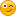Hello,

I'm currently facing the following problem: I'm trying to minimize the variance of a portfolio by changing the weights of the assets, but under a constraint regarding a different parameters, specifically the risk contribution of each asset to the total volatility of the portfolio should be equal. Now I want to introduce two constraints:

CoVarA = Covariance Matrix of Assets (4x4)
b = weight vector of the 4 assets (4x1)

1) All the weights add up to 1, which I managed with the "optimize_init_constraints" command as seen below (this one works):

Code:
mata:

mata clear

CoVarA =st_matrix("CoVarA")

void i_VarPF(todo, b, VarPF,g,H)

{

external CoVarA

b=abs(b)

VarPF = b*CoVarA*b'

}

SumConstraint=1
Unitvector=J(1,4,1)

guess=J(1,4,0)

S=optimize_init()

optimize_init_evaluator(S, &i_VarPF())

optimize_init_which(S, "min")

optimize_init_evaluatortype(S,"d0")

optimize_init_params(S, guess)

optimize_init_conv_maxiter(S, 10000)

optimize_init_constraints(S, (Unitvector,SumConstraint))

p=optimize(S)
p

end
Now to the actual problem:

2) Now the second constraint I want to introduce is not directly on the weight vector b, but on a calculated matrix including b as the only changing variable, more specifically the RelativeRiskA. This should be that each of the assets is contributing the same risk to the final portfolio, which should look like (0.25,0.25,0.25,0.25). This is the code for the calculation of the RelativeRiskA:

weights = weights of the assets --> should then here be replaced with the input from the optimization loop "b"

Code:
mata:

mata clear
FactorL = st_matrix("FactorL")
CoVarA =st_matrix("CoVarA")
weights =st_matrix("weights")

VarPF=weights'*CoVarA*weights
StdPF =sqrt(VarPF)
StdPF

MarginalRiskA=(CoVarA*weights)/(sqrt(weights'*CoVarA*weights))
MarginalRiskA

RiskContributionA=MarginalRiskA*weights'*weights
RiskContributionA

RelativeRiskA=RiskContributionA/StdPF
RelativeRiskA

end
The problem now is to put this as a constraint into the optimization function. Does anyone have an idea how to solve this issue?

Please let me know if you need any more information. I'm posting here for the first time and just been coding for a few days, so don't mind my "non-lean" codeThanks a lot for any input!!

Best regards,
Jérôme